# 6

Personal Blog

EDIT: I tested this idea in a simulation and it (un)fortunately failed.

This post is an attempt to combine some parts of frequentism with some parts of Bayesianism. Unfortunately, there is a good deal of probability theory. I assume that "improper priors" are okay to use and that we have no prior knowledge about the population of which we are trying to learn.

THEORY

Let's say we're trying to estimate some parameter, B. If we interpret the likelihood function as a conditional probability function, we get a function for our posterior probability density function after taking n samples: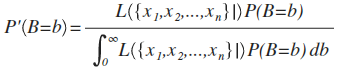This yields an expected value of B: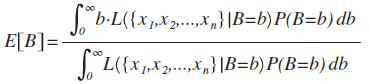Now, intuitively, it would make a lot of sense if, given B=b, the expected value of our posterior probability density function turned out to be actually be B. In frequentist terms, it would be nice if our posterior distribution was unbiased: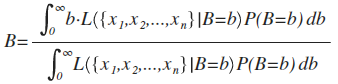So, the question is: If we're trying to estimate a parameter of some distribution, can we pick a prior distribution such that this property is true?

EXAMPLE

Let's say that we have a uniform distribution from 0 to B. So, we draw a sample of "N" numbers with some maximum value "M". Now, let's examine the hypothesis that B=b, for some "b". If b<M, we know that B=b is impossible. If b>M, we know it is possible. In particular, the likelihood function becomes: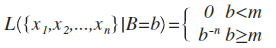The expected value calculation turns out to be: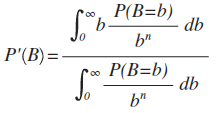Now, I leave solving this equation as an exercise for the reader, but it turns out that if we let we get an unbiased estimator for B if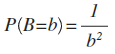Now, of course, 1/x^2 is definitely not a proper prior distribution for the simple reason that its integral is infinite. However, if we use it as an improper prior distribution, then, any sample with maximum≠0 will yield a valid probability distribution.

DISCUSSION

As far as I know there is no law of probability that says our posterior probability function "should" have an expected value equal to the parameter. That being said it does seem like a positive trait for the function to have. I also suspect that this idea can be extended to a wide variety of distributions. That being said, we obviously tend to have  prior information in real life about our populations, so the practical effects of this idea are probably minimal.

# 6

New Comment

I also think that it would be a positive trait for a function to say that there is a finite positive probability of randomly picking more than one in 3^^^3.

I don't see why prior probabilities specifically should have this property. If it was a good property, I'd expect I'd still have it even after establishing a probability distribution. Since I know I won't, I tend to think the entire expectation that it should work that way is just some sort of misunderstanding of probability. Specifically, it reminds me of the base rate fallacy. You should be worried about the expected value of the variable given your belief, not the expected value of your belief given the variable.

I had typed up an eloquent reply to address these issues, but instead wrote a program that scored uniform priors vs 1/x^2 priors for this problem. (Un)fortunately, my idea does consistently (slightly) worse using the p*log(p) metric. So, you are correct in your skepticism. Thank you for the feeback!

Here's another interesting example.

Suppose you're going to observe Y in order to infer some parameter X. You know that `P(x=c | y) = 1/2^(c-y)`.

• You set your prior to P(x=c) = 1 for all c. Very improper.
• You make an observation, y=1.
• You update: P(x=c) = 1/2^(c-1)
• You can now normalize P(x) so its area under the curve is 1.
• You could have done that, regardless of what you observed y to be. Your posterior is guaranteed to be well formed.

You get well formed probabilities out of this process. It converges to the same result that Bayesianism does as more observations are made. The main constraint imposed is that the prior must "sufficiently disagree" in predictions about a coming observation, so that the area becomes finite in every case.

I think you can also get these improper priors by running the updating process backwards. Some posteriors are only accessible via improper priors.

This is neat, thanks for posting it.